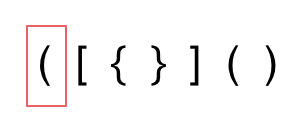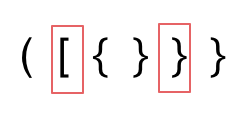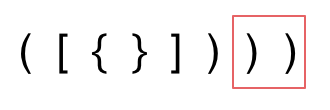# # 20. 有效的括号

• 左括号必须用相同类型的右括号闭合。
• 左括号必须以正确的顺序闭合。
• 注意空字符串可被认为是有效字符串。

• 输入: "()"
• 输出: true

• 输入: "()[]{}"
• 输出: true

• 输入: "(]"
• 输出: false

• 输入: "([)]"
• 输出: false

• 输入: "{[]}"
• 输出: true

# # 思路

## # 题外话

``````cd a/b/c/../../
``````
1

## # 进入正题

1. 第一种情况，字符串里左方向的括号多余了 ，所以不匹配。2. 第二种情况，括号没有多余，但是 括号的类型没有匹配上。3. 第三种情况，字符串里右方向的括号多余了，所以不匹配。``````class Solution {
public:
bool isValid(string s) {
stack<int> st;
for (int i = 0; i < s.size(); i++) {
if (s[i] == '(') st.push(')');
else if (s[i] == '{') st.push('}');
else if (s[i] == '[') st.push(']');
// 第三种情况：遍历字符串匹配的过程中，栈已经为空了，没有匹配的字符了，说明右括号没有找到对应的左括号 return false
// 第二种情况：遍历字符串匹配的过程中，发现栈里没有我们要匹配的字符。所以return false
else if (st.empty() || st.top() != s[i]) return false;
else st.pop(); // st.top() 与 s[i]相等，栈弹出元素
}
// 第一种情况：此时我们已经遍历完了字符串，但是栈不为空，说明有相应的左括号没有右括号来匹配，所以return false，否则就return true
return st.empty();
}
};
``````
1
2
3
4
5
6
7
8
9
10
11
12
13
14
15
16
17

## # 其他语言版本

Java：

``````class Solution {
public boolean isValid(String s) {
char ch;
for (int i = 0; i < s.length(); i++) {
ch = s.charAt(i);
//碰到左括号，就把相应的右括号入栈
if (ch == '(') {
deque.push(')');
}else if (ch == '{') {
deque.push('}');
}else if (ch == '[') {
deque.push(']');
} else if (deque.isEmpty() || deque.peek() != ch) {
return false;
}else {//如果是右括号判断是否和栈顶元素匹配
deque.pop();
}
}
//最后判断栈中元素是否匹配
return deque.isEmpty();
}
}
``````
1
2
3
4
5
6
7
8
9
10
11
12
13
14
15
16
17
18
19
20
21
22
23

Python：

``````# 方法一，仅使用栈，更省空间
class Solution:
def isValid(self, s: str) -> bool:
stack = []

for item in s:
if item == '(':
stack.append(')')
elif item == '[':
stack.append(']')
elif item == '{':
stack.append('}')
elif not stack or stack[-1] != item:
return False
else:
stack.pop()

return True if not stack else False
``````
1
2
3
4
5
6
7
8
9
10
11
12
13
14
15
16
17
18
``````# 方法二，使用字典
class Solution:
def isValid(self, s: str) -> bool:
stack = []
mapping = {
'(': ')',
'[': ']',
'{': '}'
}
for item in s:
if item in mapping.keys():
stack.append(mapping[item])
elif not stack or stack[-1] != item:
return False
else:
stack.pop()
return True if not stack else False
``````
1
2
3
4
5
6
7
8
9
10
11
12
13
14
15
16
17

Go：

``````func isValid(s string) bool {
hash := map[byte]byte{')':'(', ']':'[', '}':'{'}
stack := make([]byte, 0)
if s == "" {
return true
}

for i := 0; i < len(s); i++ {
if s[i] == '(' || s[i] == '[' || s[i] == '{' {
stack = append(stack, s[i])
} else if len(stack) > 0 && stack[len(stack)-1] == hash[s[i]] {
stack = stack[:len(stack)-1]
} else {
return false
}
}
return len(stack) == 0
}
``````
1
2
3
4
5
6
7
8
9
10
11
12
13
14
15
16
17
18

Ruby:

``````def is_valid(strs)
symbol_map = {')' => '(', '}' => '{', ']' => '['}
stack = []
strs.size.times {|i|
c = strs[i]
if symbol_map.has_key?(c)
top_e = stack.shift
return false if symbol_map[c] != top_e
else
stack.unshift(c)
end
}
stack.empty?
end
``````
1
2
3
4
5
6
7
8
9
10
11
12
13
14

Javascript:

``````var isValid = function (s) {
const stack = [];
for (let i = 0; i < s.length; i++) {
let c = s[i];
switch (c) {
case '(':
stack.push(')');
break;
case '[':
stack.push(']');
break;
case '{':
stack.push('}');
break;
default:
if (c !== stack.pop()) {
return false;
}
}
}
return stack.length === 0;
};
// 简化版本
var isValid = function(s) {
const stack = [],
map = {
"(":")",
"{":"}",
"[":"]"
};
for(const x of s) {
if(x in map) {
stack.push(x);
continue;
};
if(map[stack.pop()] !== x) return false;
}
return !stack.length;
};
``````
1
2
3
4
5
6
7
8
9
10
11
12
13
14
15
16
17
18
19
20
21
22
23
24
25
26
27
28
29
30
31
32
33
34
35
36
37
38
39